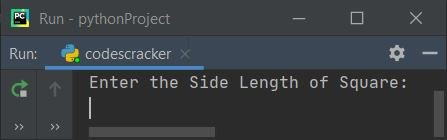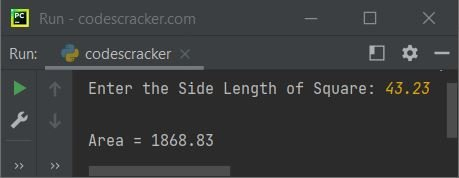# Python Program to Find Area of Square

This article is created to cover some programs in Python, to find and print area of a square based on the length of its side, entered by user at run-time. Here are the list of programs:

• Find Area of Square without Function
• Find Area of Square using User-defined Function
• Using Class and Object

### Formula to Find Area of Square

To find area of a square, use:

```area = len2
= len*len```

Here area indicates to area value and len indicates to length value of square

## Find Area of Square

To find area of a square in Python, you have to ask from user to enter the side length. Now use the formula to find and print the area value as shown in the program given below:

```print("Enter the Side Length of Square: ")
l = float(input())
a = l*l
print("\nArea = ", a)```

Here is the initial output produced by this program:Now supply the input say 6 as side length of square to find and print its area based on the given value of its side length as shown in the snapshot given below:## Find Area of Square using Function

This program is created using a user-defined function named areaOfSquare(). This function takes side length as its argument and returns the area value. The return value of this function gets initialized to a. Now print the value of a as output. That's it:

```def areaOfSquare(s):
return s*s

print("Enter the Side Length of Square: ", end="")
l = float(input())
a = areaOfSquare(l)
print("\nArea = {:.2f}".format(a))```

Here is its sample run with user input, 43.23 as side length of square:## Find Area of Square using Class

This is the last program on finding area of a square, using class and object, an object-oriented feature of Python. The program is little similar to the program created using function. The only difference is, we've to call the member function areaOfSquare() of class using its object through dot (.) operator.

Note - Before accessing any member function of a class using an object say obj, the properties of class must be assigned to it. Therefore through the statement, obj = CodesCracker(), we've done the job.

```class CodesCracker:
def areaOfSquare(self, s):
return s*s

print("Enter the Side Length of Square: ", end="")
l = float(input())

obj = CodesCracker()
a = obj.areaOfSquare(l)

print("\nArea = {:.2f}".format(a))```

This program produces the same output as of previous program.

#### Same Program in Other Languages

Python Online Test

« Previous Program Next Program »